## A professor wants to estimate the average time it takes his students to finish a computer project. Based on previous evidence, he believes t

Question

A professor wants to estimate the average time it takes his students to finish a computer project. Based on previous evidence, he believes that the standard deviation is approximately 3.6 hours. He would like to be 96% confident that his estimate is within 5 hours of the true population mean. Use RStudio to determine how large of a sample size is required without rounding any interim calculations.

in progress 0
6 months 2021-08-27T04:30:36+00:00 1 Answers 4 views 0

A sample size of 3 is required.

Step-by-step explanation:

We have that to find our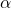level, that is the subtraction of 1 by the confidence interval divided by 2. So: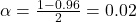Now, we have to find z in the Z-table as such z has a p-value of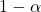.

That is z with a pvalue of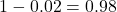, so Z = 2.054.

Now, find the margin of error M as such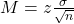In which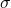is the standard deviation of the population and n is the size of the sample.

Based on previous evidence, he believes that the standard deviation is approximately 3.6 hours.

This means that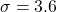He would like to be 96% confident that his estimate is within 5 hours of the true population mean. Use RStudio to determine how large of a sample size is required without rounding any interim calculations.

The sample size needed is of n, and n is found when M = 5. So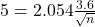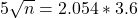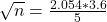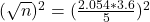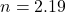Rounding up:

A sample size of 3 is required.